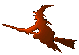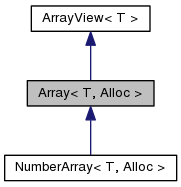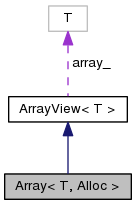Hex  1.0 Hydrogen-electron collision solver
Array< T, Alloc > Class Template Reference

A comfortable data array class. More...

#include <arrays.h>

Inheritance diagram for Array< T, Alloc >:[legend]
Collaboration diagram for Array< T, Alloc >:[legend]

## Public Types

typedef T DataType

typedef T * iterator

typedef T const * const_iteratorPublic Types inherited from ArrayView< T >
typedef T DataType

typedef T * iterator

typedef T const * const_iterator

## Public Member Functions

Array ()

Alloc::alloc (n))

Array (size_t n, T const *const x)

Array (Array< T > const &a)

Array (const ArrayView< T > a)

Array (Array< T > &&a)

Array (std::vector< T > const &a)

Array (std::initializer_list< T > a)

template<typename ForwardIterator >
Array (ForwardIterator i, ForwardIterator j)

~Array ()

size_t size () const
Return number of elements. More...

virtual size_t resize (size_t n)
Resize array. More...

T & operator[] (size_t i)
Element-wise access (non-const). More...

T const & operator[] (size_t i) const
Element-wise access (const). More...

iterator begin ()

const_iterator begin () const

iterator end ()

const_iterator end () const

T & front (int i=0)

T const & front (int i=0) const

T & back (int i=0)

T const & back (int i=0) const

virtual void push_back (T const &a)
Append an element to the end. More...

virtual T pop_back ()
Remove the last element from the array. More...

template<class InputIterator >
void append (InputIterator first, InputIterator last)
Append more items. More...

void insert (iterator it, T x)
Insert item. More...

bool empty () const
Check that size equals to zero. More...

Array< T > & operator= (Array< T > const &b)

Array< T > & operator= (Array< T > &&b)

virtual T * data ()
Data pointer. More...

virtual T const * data () constPublic Member Functions inherited from ArrayView< T >
ArrayView ()

ArrayView (size_t n, T *ptr)

ArrayView (ArrayView< T > const &a, size_t i=0, size_t n=0)

ArrayView (Array< T > const &a, size_t i=0, size_t n=0)

ArrayView (NumberArray< T > const &a, size_t i=0, size_t n=0)

ArrayView (const_iterator i, const_iterator j)

ArrayView (ArrayView< T > &&r)

virtual ~ArrayView ()

ArrayView< T > & operator= (const ArrayView< T > v)
Assignment operator. More...

T & operator[] (size_t i)
Element-wise access (non-const). More...

T const & operator[] (size_t i) const
Element-wise access (const). More...

size_t size () const
Length of the array (number of elements). More...

iterator begin ()

const_iterator begin () const

iterator end ()

const_iterator end () const

T & front (int i=0)

T const & front (int i=0) const

T & back (int i=0)

T const & back (int i=0) const

void fill (T x)
Fill the array with a value. More...

bool empty () const
Check whether the size is equal to zero. More...

template<class = typename std::enable_if<is_scalar<T>::value>>
double norm () const
Two-norm (defined only for scalar data type). More...

## Data Fields

__pad0__: ArrayView<T>(n

## Additional Inherited MembersProtected Attributes inherited from ArrayView< T >
size_t N_
Number of elements in the array. More...

T * array_
Pointer to the array. More...

## Detailed Description

### template<class T, class Alloc = PlainAllocator<T>> class Array< T, Alloc >

Class Array is intended as a Hex's replacement for std::vector<T>. Properties:

• User specified allocator (given as the second template argument of the class). See PlainAllocator and AlignedAllocator for examples of such allocators. Allocator is expected to have two static methods: void* alloc(size_t) that returns a pointer to new memory chunk and void free() which deallocates the pointer.
• Basic iterator interface simillar to STL containers – methods begin(), end(), and thus also the ability to appear in the range-based for loops.

## Member Typedef Documentation

template<class T, class Alloc = PlainAllocator<T>>
 typedef T const* Array< T, Alloc >::const_iterator
template<class T, class Alloc = PlainAllocator<T>>
 typedef T Array< T, Alloc >::DataType
template<class T, class Alloc = PlainAllocator<T>>
 typedef T* Array< T, Alloc >::iterator

## Constructor & Destructor Documentation

template<class T, class Alloc = PlainAllocator<T>>
 Array< T, Alloc >::Array ( )
inline
template<class T, class Alloc = PlainAllocator<T>>
 Array< T, Alloc >::Array ( size_t n, T const *const x )
inline
template<class T, class Alloc = PlainAllocator<T>>
 Array< T, Alloc >::Array ( Array< T > const & a )
inline
template<class T, class Alloc = PlainAllocator<T>>
 Array< T, Alloc >::Array ( const ArrayView< T > a )
inline
template<class T, class Alloc = PlainAllocator<T>>
 Array< T, Alloc >::Array ( Array< T > && a )
inline
template<class T, class Alloc = PlainAllocator<T>>
 Array< T, Alloc >::Array ( std::vector< T > const & a )
inline
template<class T, class Alloc = PlainAllocator<T>>
 Array< T, Alloc >::Array ( std::initializer_list< T > a )
inline
template<class T, class Alloc = PlainAllocator<T>>
template<typename ForwardIterator >
 Array< T, Alloc >::Array ( ForwardIterator i, ForwardIterator j )
inline
template<class T, class Alloc = PlainAllocator<T>>
 Array< T, Alloc >::~Array ( )
inline

## Member Function Documentation

template<class T, class Alloc = PlainAllocator<T>>
 Array< T, Alloc >::Alloc::alloc ( n )
inline
template<class T, class Alloc = PlainAllocator<T>>
template<class InputIterator >
 void Array< T, Alloc >::append ( InputIterator first, InputIterator last )
inline

The function appends a range of items to the end of the array. A reallocation takes place. The range is specified using input iterators.

template<class T, class Alloc = PlainAllocator<T>>
 T& Array< T, Alloc >::back ( int i = 0 )
inline
template<class T, class Alloc = PlainAllocator<T>>
 T const& Array< T, Alloc >::back ( int i = 0 ) const
inline
template<class T, class Alloc = PlainAllocator<T>>
 iterator Array< T, Alloc >::begin ( )
inline
template<class T, class Alloc = PlainAllocator<T>>
 const_iterator Array< T, Alloc >::begin ( ) const
inline
template<class T, class Alloc = PlainAllocator<T>>
 virtual T* Array< T, Alloc >::data ( )
inlinevirtual

Reimplemented from ArrayView< T >.

template<class T, class Alloc = PlainAllocator<T>>
 virtual T const* Array< T, Alloc >::data ( ) const
inlinevirtual

Reimplemented from ArrayView< T >.

template<class T, class Alloc = PlainAllocator<T>>
 bool Array< T, Alloc >::empty ( ) const
inline
template<class T, class Alloc = PlainAllocator<T>>
 iterator Array< T, Alloc >::end ( )
inline
template<class T, class Alloc = PlainAllocator<T>>
 const_iterator Array< T, Alloc >::end ( ) const
inline
template<class T, class Alloc = PlainAllocator<T>>
 T& Array< T, Alloc >::front ( int i = 0 )
inline
template<class T, class Alloc = PlainAllocator<T>>
 T const& Array< T, Alloc >::front ( int i = 0 ) const
inline
template<class T, class Alloc = PlainAllocator<T>>
 void Array< T, Alloc >::insert ( iterator it, T x )
inline

Function "insert" inserts new item "x" to the array at position pointed to by the iterator "it". Reallocation always taked place. If the iterator points to the end of the original array, the effect is the same as push_back. Otherwise, the items behind the iterator are shifted to make place for the new item.

template<class T, class Alloc = PlainAllocator<T>>
 Array& Array< T, Alloc >::operator= ( Array< T > const & b )
inline
template<class T, class Alloc = PlainAllocator<T>>
 Array& Array< T, Alloc >::operator= ( Array< T > && b )
inline
template<class T, class Alloc = PlainAllocator<T>>
 T& Array< T, Alloc >::operator[] ( size_t i )
inline
template<class T, class Alloc = PlainAllocator<T>>
 T const& Array< T, Alloc >::operator[] ( size_t i ) const
inline
template<class T, class Alloc = PlainAllocator<T>>
 virtual T Array< T, Alloc >::pop_back ( )
inlinevirtual

The last element of the array will be ignored, which is achieved by decrementing array length. The memory will not be deallocated to save processor time. For this reason, if an array is "erased" by subsequent calls to pop_back, it will still occupy the same memory as before. The deallocation will take place only on resize.

template<class T, class Alloc = PlainAllocator<T>>
 virtual void Array< T, Alloc >::push_back ( T const & a )
inlinevirtual

The function will append the element "a" to the end of the array. This will require allocation of a longer array and copy of the original elements to the new array (using the rvalue reference). The function is declared as virtual so that it can be safely overridden in derived classes.

template<class T, class Alloc = PlainAllocator<T>>
 virtual size_t Array< T, Alloc >::resize ( size_t n )
inlinevirtual

The method will change the length of the array, most probably by reallocating the storage. The possible new elements will be initialized to T(0). The function is virtual, so that is can be safely overridden in the derived classes.

template<class T, class Alloc = PlainAllocator<T>>
 size_t Array< T, Alloc >::size ( ) const
inline

## Field Documentation

template<class T, class Alloc = PlainAllocator<T>>
 Array< T, Alloc >::__pad0__

The documentation for this class was generated from the following file: